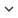ENGCongress
Congress 938
Author/s
• Ismael Rodríguez-Fdez, Manuel Mucientes and Alberto Bugarín
DOI
Source
• 2015 IEEE International Conference on Fuzzy Systems. Estambul, Turquía. 2015

# Reducing the Complexity in Genetic Learning of Accurate Regression TSK Rule-Based Systems

In many real problems the regression models have to be accurate but, also, interpretable in order to provide qualitative understanding of the system. In this realm, the use of fuzzy rule base systems, particularly TSK, is widely extended. TSK rules combine the interpretability and expressiveness of rules with the ability of fuzzy logic for representing uncertainty, and the precision of the polynomials in the consequents. In this paper we present a new genetic fuzzy system to automatically learn accurate and simple linguistic TSK fuzzy rule bases that accurately model regression problems. In order to reduce the complexity of the learned models while keeping a high accuracy, we propose a Genetic Fuzzy System which consists of three stages: instance selection, multi-granularity fuzzy discretization of the input variables, and the evolutionary learning of the rule base using Elastic Net regularization. This proposal was validated using 28 real-world datasets and compared with three state of the art genetic fuzzy systems. Results show that our approach obtains the simplest models while achieving a similar accuracy to the best approximative models.
Keywords: Genetic Fuzzy Systems, regression, instances selection, multi-granularity fuzzy discretization